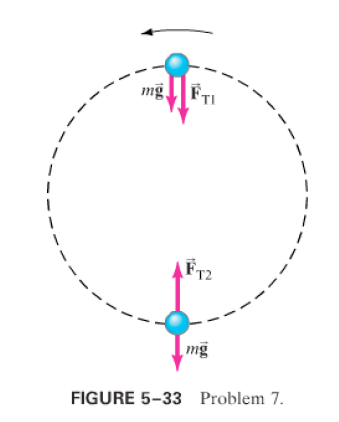×
Get Full Access to Physics: Principles With Applications - 6 Edition - Chapter 5 - Problem 7p
Get Full Access to Physics: Principles With Applications - 6 Edition - Chapter 5 - Problem 7p

×

# A ball on the end of a string is revolved at a uniformISBN: 9780130606204 3

## Solution for problem 7P Chapter 5

Physics: Principles with Applications | 6th Edition

• Textbook Solutions
• 2901 Step-by-step solutions solved by professors and subject experts
• Get 24/7 help from StudySoup virtual teaching assistantsPhysics: Principles with Applications | 6th Edition

4 5 1 286 Reviews
30
4
Problem 7P

(II) A ball on the end of a string is revolved at a uniform rate in a vertical circle of radius, as shown in Fig.If its speed isand its mass is, calculate the tension in the string when the ball isat the top of its path, andat the bottom of its path.Step-by-Step Solution:

Solution: The above figure is in equilibrium. Step 1 What is Static equilibrium : Static equilibrium is a form of equilibrium that occurs when an object is at rest. “Static” refers to the object being motionless while “equilibrium” refers to the object either having no net forces acting upon it or having all of its net forces balanced. So the above figure as an object is in static equilibrium, then it has no net torque and no net force.

Step 2 of 2

##### ISBN: 9780130606204

Unlock Textbook Solution